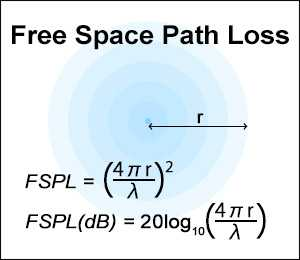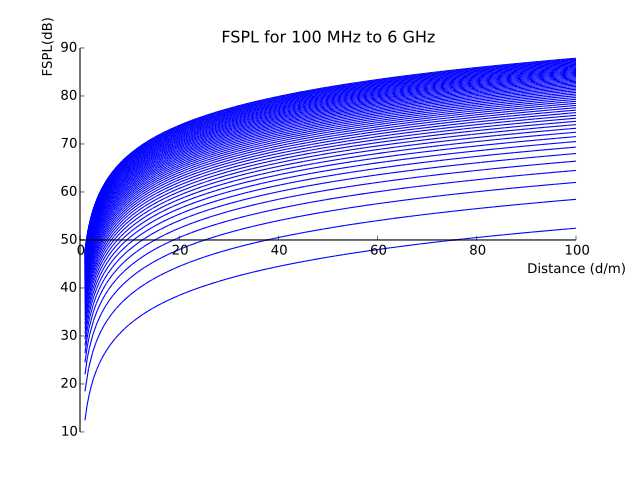# Free Space Path Loss Calculator

This calculator calculates free space path loss(FSPL).

Free space path loss is often given in decibels so this calculator calculates the decibel value of FSPL as well.

The inverse function of free space path loss is used in the Friis transmission equation.

The decibel value is rounded to the second decimal point. The speed of light is calculated as 299792458(m/s)(Only used when a frequency value is given).

Equations

FSPL = (4*π*r/λ)^2

FSPL(dB) = 10*log((4*π*r/λ)^2) = 20*log(4*π*r/λ)

λ = wavelength(m), r = distance(m)### Input

Distance(r):

Frequency(f):

Wavelength(λ):
Use frequency value
Use wavelength value

### Output"A plot of the FSPL for a range of fixed frequencies. FSPL increases with increasing frequency."

By Sss41 - Own work, CC BY-SA 3.0